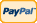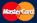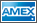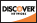•Electronics, electrical engineering
•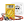Engineering physics
•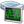Generators
•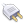Interfaces
•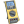Multimeters
•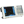Oscilloscopes
•Persona
•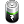Power supplies
•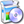Software
•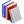Standards
•Thesaurus
•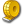Units of measurement
Filter by first letter
A
B
C
D
E
F
G
H
I
J
K
L
M
N
O
P
Q
R
S
T
U
V
W
X
Y
Z
+
-

# Newton (N)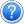The newton (symbol: N) is the SI derived unit of force. It is named after Isaac Newton in recognition of his work on classical mechanics, specifically Newton's second law of motion.

It is equal to the amount of net force required to accelerate a mass of one kilogram at a rate of one meter per second squared. Since Newton's second law of motion states F = ma, multiplying m (kg) by a (m/s2), the newton is therefore: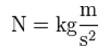www.wikipedia.org

Back to the list

Units Converter
• How it Works
• Company News
• Industry News & Events
• Measurement Fun Facts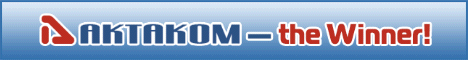Site map|Privacy policy|Terms of Use & Store Policies|How to Buy|Shipping|Payment|© T&M Atlantic, Inc., 2010-2022